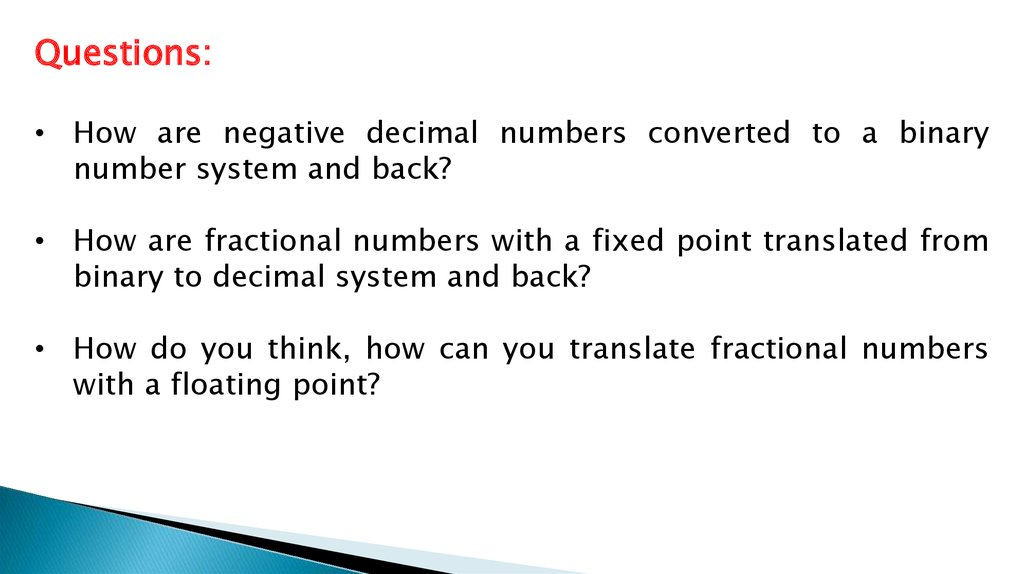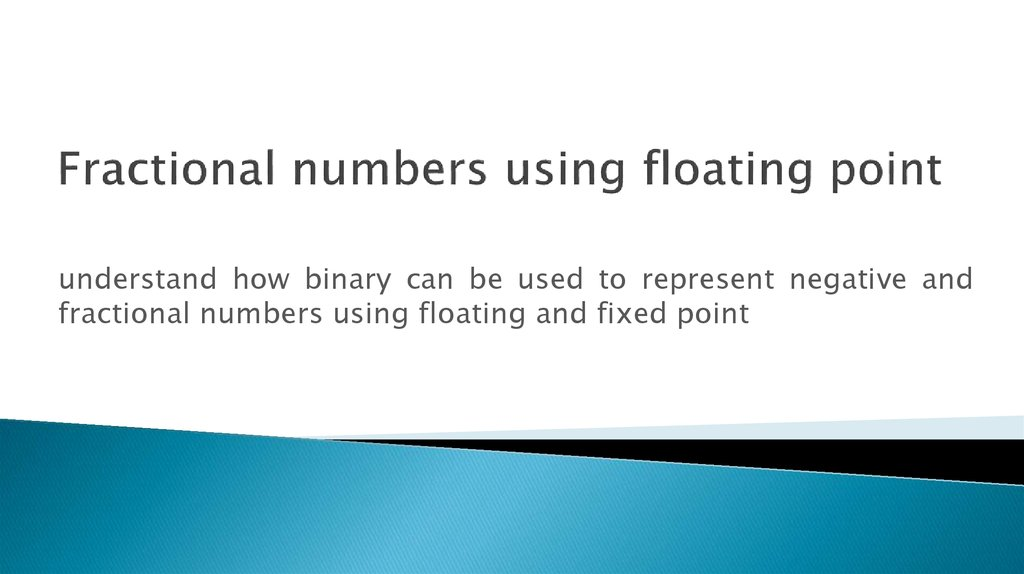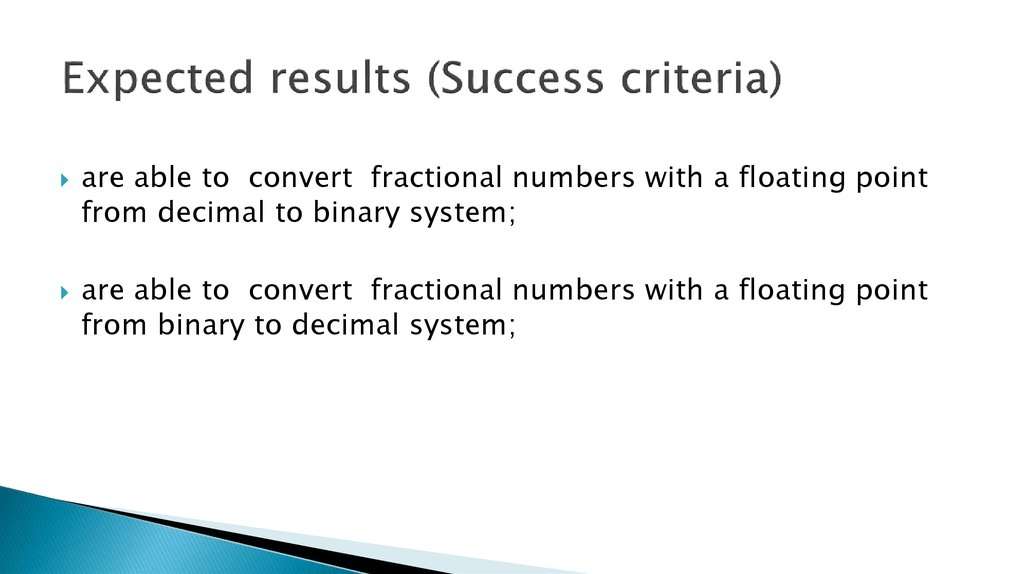# Floating point numbers

## 1.

Questions:
• How are negative decimal numbers converted to a binary
number system and back?
• How are fractional numbers with a fixed point translated from
binary to decimal system and back?
• How do you think, how can you translate fractional numbers
with a floating point?

## 2.

understand how binary can be used to represent negative and
fractional numbers using floating and fixed point

## 3. Fractional numbers using floating point

are able to convert fractional numbers with a floating point
from decimal to binary system;
are able to convert fractional numbers with a floating point
from binary to decimal system;

## 4. Expected results (Success criteria)

Fractional numbers using floating point
The first bit defines the non-zero part of the number and is called the
Mantissa, the second part defines how many positions we want to move
the decimal point, this is known as the Exponent and can be positive
when moving the decimal point to the right and negative when moving to
the left.

## 5.

Converting binary floating point to decimal
• Sign - find the sign of the mantissa (make a note of this)
• Slide - find the value of the exponent and whether it is positive or negative
• Bounce - move the decimal the distance the exponent asks, left for a negative
exponent, right for a positive
• If Moving Left and Is Positive Number, Then pad with zeroes
• If Moving Left and Is Negative Number, Then pad with ones
• Flip - If the mantissa is negative perform twos complement on it
• Swim - starting at the decimal point work out the values of the mantissa,
going left, then right. Now make sure you refer back to the sign you recorded
on the sign move.

## 6.

Exercise: Simple binary floating point
Work out the denary for the following, using 10 bits for the mantissa and 6 bits for the exponent:
0.001101000 000110
1. Sign: the mantissa starts with a zero, therefore it is a positive number.
2. Slide: work out the value of the exponent
000110 = +6
3. Bounce: we need to move the decimal point in the mantissa. In this case the
exponent was positive so we need to move the decimal point 6 places to the right
0.001101000 -> 0001101.000
4. Flip: as the number isn't negative we don't need to do this
5. Swim: work out the value on the left hand side and right hand side of the decimal
point
1+4+8 = +13

## 7.

Work out the denary for the following, using 10 bits for the mantissa and 6 bits for the exponent:
0 101000000 111111
1. Sign: the mantissa starts with a zero, therefore it is a positive number.
2. Slide: work out the value of the exponent
111111 It starts with a one therefore it is a
negative number 000001 = -1
3. Bounce: we need to move the decimal point in the mantissa. In this case the
exponent was negative so we need to move the decimal point 1 place to the left
0.101000000 -> 0.0101000000
4. Flip: as the mantissa number isn't negative we don't need to do this
5. Swim: work out the value on the left hand side and right hand side of the decimal
point
1/4 + 1/16 = +0.3125

## 8.

Work out the denary for the following, using 10 bits for the mantissa and 6 bits for the
exponent:
1 011111010 000101
1. Sign: the mantissa starts with a one, therefore it is a negative number.
2. Slide: work out the value of the exponent
000101 = +5
3. Bounce: we need to move the decimal point in the mantissa. In this case the
exponent was positive so we need to move the decimal point 5 places to the right
1.011111010 -> 101111.1010
4. Flip: the mantissa is negative as noted in step one so we need to convert this
number
101111.1010 -> 010000.0110
5. Swim: work out the value on the left hand side and right hand side of the decimal
point
16+1/4+1/8 = -16.375 FINISHED!

## 9.

Example: denary to binary floating point
If we are asked to convert the denary number 39.75 into binary floating point we first need to find out
the binary equivalent:
128 64 32 16 8 4 2 1 . ½ ¼ ⅛
0
0 1 0 0 1 1 1 . 1 1 0
How far do we need to move the binary point to the left so that the number is normlised?
0 0 . 1 0 0 1 1 1 1 1 0 (6 places to the left)
So to get our decimal point back to where it started, we need to move 6 places to the right. 6 now
0.100111110 | 000110
If you want to check your answer, convert the number above into decimal. You get 39.75!

## 10.

Work out the binary floating point for the following, using 10 bits for the mantissa and
6 bits for the exponent: 67
128 64 32 16 8 4 2 1 . ½ ¼ ⅛
0 1 0
0 0 0 1 1 . 0 0 0
How far do we need to move the binary point to the left so that the number is normlised?
0 . 1 0 0 0 0 1 1 0 0 0 (7 places to the left)
To get the front to be normalised we must move the decimal point 7 places.
0.100001100 | 000111

## 11.

Work out the binary floating point for the following, using 10 bits for the mantissa
and 6 bits for the exponent: 23.25
128 64 32 16 8 4 2 1 . ½ ¼ ⅛
0
0 0 1 0 1 1 1 . 0 1 0
How far do we need to move the binary point to the left so that the number is
normlised?
0 0 0 . 1 0 1 1 1 0 1 0 (5 places to the left)
To get the front to be normalised we must move the decimal point 5 places.
0.101110100 | 000101

## 12.

Work out the denary for the following, using 10 bits for the mantissa and 6 bits for the
exponent:
1) 1 111111010 000011
2) 1 011111010 000101
Work out the binary floating point for the following, using 10 bits for the mantissa and
6 bits for the exponent:
1) 123.875
2) 128.25
3) 29.75

## 13.

1) 1/16+1/32 = -0.09375
2) -16.375
1) 1/2+1/8 +1/16 = -0.34375
2) -20.875 (16+4.1/2+1/4+1/8)
1) 0.111101111 | 000111
2) 0.100000000 | 001000
3) 0,111011100 | 000101
1) 0.111100111 | 001000
2) 0.100011010 | 001001

## 14.

• What is the difference between fractional numbers
with a floating point and fractional numbers with a
fixed point?
• How to translate fractional numbers with a floating
point from the decimal system to binary and back?